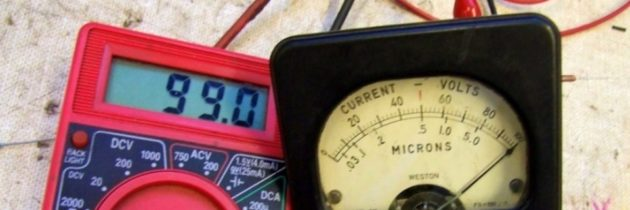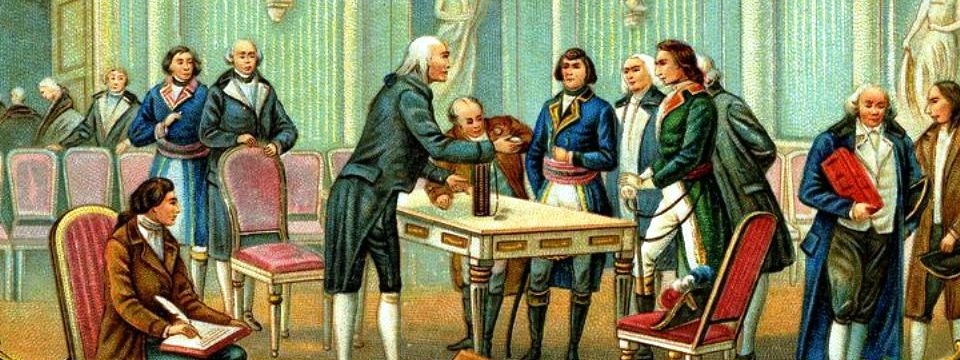Posted by on Nov 13, 2017 in Tell Me Why Numerous Questions and Answers |# What Is a Volt?

A volt is the practical unit of electromotive force, or electrical pressure, as distinct from an electric current. In terms of measurement it is that electrical motive force which applied to a conductor of 1 ohm resistance produces a current of 1 ampere.

Imagine water stored in a tank on a tower, with an outlet near the ground. The head of water will give varying pressures at ground level, depending on the height of the tower. In electricity the head of water corresponds to the voltage of the supply.The volt is named after the Italian physicist Alexandro Volta (1745-1827), who invented the voltaic cell. In 1800, he built the voltaic pile and discovered the first practical method of generating electricity. Count Volta also made discoveries in electrostatics, meteorology and pneumatics. His most famous invention, however, is the first battery.

In a dry battery the chemical composition determines the voltage or pressure of each cell. This cannot be increased by making the cell larger. A larger cell will merely last longer.

Volts and amperes are measures of electricity. A volt is the unit of electric potential difference, or the size of the force that sends the electrons through a circuit.

One Volt is defined as energy consumption of one joule per electric charge of one coulomb.

1V = 1J/C

One volt is equal to current of 1 amp time’s resistance of 1 ohm:

1V = 1A ⋅ 1Ω

An ampere is the unit used to measure electric current. Current is a count of the number of electrons flowing through a circuit. One amp is the amount of current produced by a force of one volt acting through the resistance of one ohm.

(An ohm is a way of measuring resistance. A certian length of copper wire, which is a good conductor, has a resistance of .0000017 ohms, while the same length of Sulfur, which is a very poor conductor, is much more resistant. It has a resistance of 200,000,000,000,000,000 ohms!)Content for this question contributed by Melissa Yonkers, resident of Rothbury, Grant Township, Oceana County, Michigan, USA